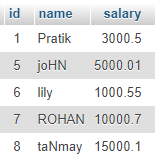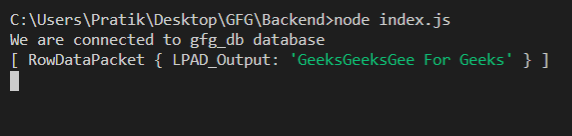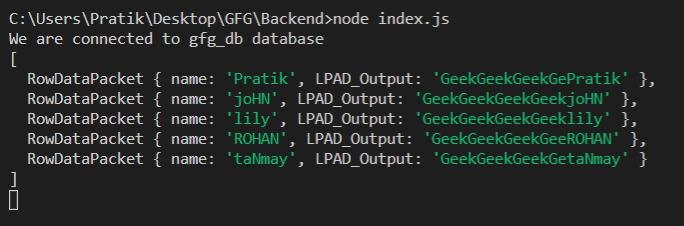Related Articles
• Last Updated : 18 Feb, 2021

LPAD() Function is a Builtin function in MySQL which is used to attach a given string to text from left side till total length is equal to given value. If the given string is small then it is repeated multiple times.

Syntax:

`LPAD(text, total_length, string)`

Parameters: LPAD() function accepts three parameters as mentioned above and described below.

• text: In this text value will be padded from left side
• total_length: Total length of output string
• string: This string value will be padded from left side

Return Value: LPAD() function returns a string by attaching value to text from left side till total length is equal to given value. If the value is small then it is repeated multiple times.

Modules:

• mysql: To handle MySQL Connection and Queries
`npm install mysql`

SQL publishers Table Preview:Example 1:

## Javascript

 `const mysql = require(``"mysql"``);`` ` `let db_con = mysql.createConnection({``  ``host: ``"localhost"``,``  ``user: ``"root"``,``  ``password: ``""``,``  ``database: ``"gfg_db"``,``});`` ` `db_con.connect((err) => {``  ``if` `(err) {``    ``console.log(``"Database Connection Failed !!!"``, err);``    ``return``;``  ``}`` ` `  ``console.log(``"We are connected to gfg_db database"``);`` ` `  ``// here is the query``  ``let query = `SELECT LPAD(``' For Geeks'``, 23, ``'Geeks'``) AS LPAD_Output`;`` ` `  ``db_con.query(query, (err, rows) => {``    ``if` `(err) ``throw` `err;`` ` `    ``console.log(rows);``  ``});``});`

Output:Example 2:

## Javascript

 `const mysql = require(``"mysql"``);`` ` `let db_con = mysql.createConnection({``  ``host: ``"localhost"``,``  ``user: ``"root"``,``  ``password: ``""``,``  ``database: ``"gfg_db"``,``});`` ` `db_con.connect((err) => {``  ``if` `(err) {``    ``console.log(``"Database Connection Failed !!!"``, err);``    ``return``;``  ``}`` ` `  ``console.log(``"We are connected to gfg_db database"``);`` ` `  ``// here is the query``  ``let query = `SELECT name, LPAD(name, 20, ``'Geek'``) ``               ``AS LPAD_Output FROM publishers`;`` ` `  ``db_con.query(query, (err, rows) => {``    ``if` `(err) ``throw` `err;`` ` `    ``console.log(rows);``  ``});``});`

Output:My Personal Notes arrow_drop_up